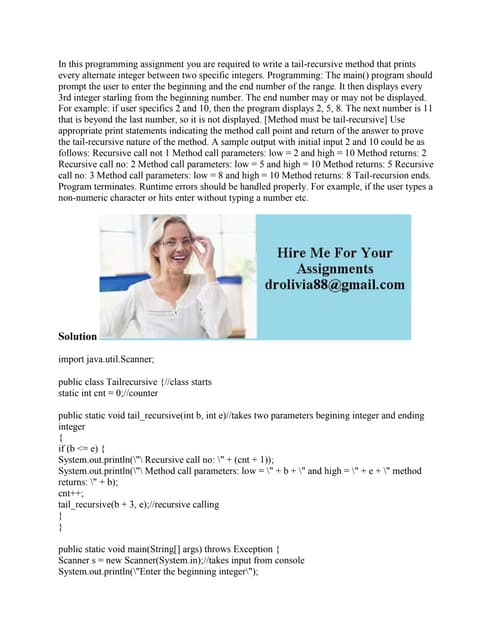PublicitéProchain SlideShareIn this programming assignment you are required to write a tail-recurs.docx
Chargement dans ... 3
1 sur 1
Publicité

### Write a program using C language- (not C++!!) that can serve as a simp.docx

1. Write a program using C language, (not C++!!) that can serve as a simple calculator. This calculator keeps track of a single number (of type double) that is called result and that starts out as 0.0. Each cycle allows the user to repeatedly add, subtract, multiply, or divide by a second number. The result of one of these operations becomes the new value of result. The calculation ends when the user enters the letter R for â€œresultâ€• (either in uppercase or lowercase). The user is allowed to do another calculation from the beginning as often as he or she wants. Use the scanf for input. The input format is shown in the following sample dialog. If the user enters any operator symbol other than +, , *, or /, then display message â€œUnknownOperatorException is thrown â€œ and the user is asked to reenter that line of input.. Calculator is on. result = 0.0 +5 result + 5.0 = 5.0 result = 5.0 *2.2 result * 2.2 = 11.0 result = 11.0 % 10 % is an unknown operation Reenter, your last line: * 0.1 result * 0.1 = 1.1 result = 1.1 r Final result = 1.1 Again? (y/n) yes result = 0.0 +10 result + 10.0 =10.0 result = 10.0 /2 result / 2.0 = 5.0 updated result = 5.0 r Final result = 5.0 Again? (y/n) N End of Program Solution # include int main() { char o; float num1,num2; printf("Enter operator either + or - or * or divide : "); scanf("%c",&o); printf("Enter two operands: "); scanf("%f%f",&num1,&num2); switch(o) { case '+': printf("%.1f + %.1f = %.1f",num1, num2, num1+num2); break; case '-': printf("%.1f - %.1f = %.1f",num1, num2, num1-num2); break; case '*': printf("%.1f * %.1f = %.1f",num1, num2, num1*num2); break; case '/': printf("%.1f / %.1f = %.1f",num1, num2, num1/num2); break; default: /* If operator is other than +, -, * or /, error message is shown */ printf("Error! operator is not correct"); break; } return 0; }
Publicité